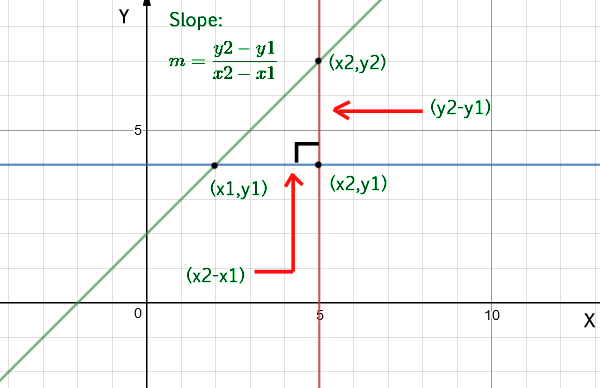# Program to find slope of a line

Given two co-ordinates, find the slope of a straight line.

Examples:

```Input  : x1 = 4, y1 = 2,
x2 = 2, y2 = 5
Output : Slope is -1.5
```

## Recommended: Please try your approach on {IDE} first, before moving on to the solution.

Approach: To calculate the slope of a line you need only two points from that line, (x1, y1) and (x2, y2). The equation used to calculate the slope from two points is:Below is the implementation of the above approach:

## c++

 `// C program for slope of line ` `#include ` `using` `namespace` `std; ` ` `  `// function to find the slope of a straight line ` `float` `slope(``float` `x1, ``float` `y1, ``float` `x2, ``float` `y2) ` `{ ` `    ``return` `(y2 - y1) / (x2 - x1); ` `} ` ` `  `// driver code to check the above function ` `int` `main() ` `{ ` `    ``float` `x1 = 4, y1 = 2; ` `    ``float` `x2 = 2, y2 = 5; ` `    ``cout << ``"Slope is: "`  `         ``<< slope(x1, y1, x2, y2); ` `    ``return` `0; ` `} `

## Java

 `// Java program for slope of line ` `import` `java.io.*; ` ` `  `class` `GFG { ` `    ``static` `float` `slope(``float` `x1, ``float` `y1, ` `                       ``float` `x2, ``float` `y2) ` `    ``{ ` `        ``return` `(y2 - y1) / (x2 - x1); ` `    ``} ` `    ``public` `static` `void` `main(String[] args) ` `    ``{ ` `        ``float` `x1 = ``4``, y1 = ``2``; ` `        ``float` `x2 = ``2``, y2 = ``5``; ` `        ``System.out.println(``"Slope is "` `+  ` `                    ``slope(x1, y1, x2, y2)); ` `    ``} ` `} `

## Python

 `# Python program for slope of line ` `def` `slope(x1, y1, x2, y2): ` `    ``return` `(``float``)(y2``-``y1)``/``(x2``-``x1)  ` ` `  `# driver code     ` `x1 ``=` `4` `y1 ``=` `2`  `x2 ``=` `2`  `y2 ``=` `5`  `print` `"Slope is :"``, slope(x1, y1, x2, y2) `

## C#

 `// C# program for slope of line ` `using` `System; ` ` `  `class` `GFG  ` `{ ` `    ``static` `float` `slope(``float` `x1, ``float` `y1, ` `                       ``float` `x2, ``float` `y2) ` `    ``{ ` `        ``return` `(y2 - y1) / (x2 - x1); ` `    ``} ` `     `  `    ``// Driver code ` `    ``public` `static` `void` `Main() ` `    ``{ ` `        ``float` `x1 = 4, y1 = 2; ` `        ``float` `x2 = 2, y2 = 5; ` `        ``Console.WriteLine(``"Slope is "` `+  ` `                    ``slope(x1, y1, x2, y2)); ` `    ``} ` `} ` ` `  `// This code is contributed by vt_m. `

## PHP

 ` `

Output:

```Slope is: -1.5
```

My Personal Notes arrow_drop_upCheck out this Author's contributed articles.

If you like GeeksforGeeks and would like to contribute, you can also write an article using contribute.geeksforgeeks.org or mail your article to contribute@geeksforgeeks.org. See your article appearing on the GeeksforGeeks main page and help other Geeks.

Please Improve this article if you find anything incorrect by clicking on the "Improve Article" button below.

Improved By : vt_m

Article Tags :
Practice Tags :

Be the First to upvote.

Please write to us at contribute@geeksforgeeks.org to report any issue with the above content.